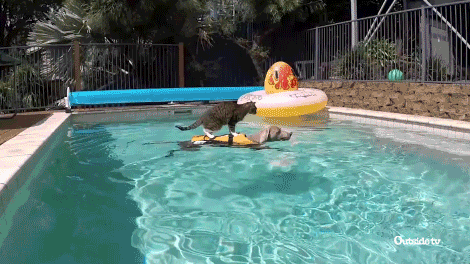# As Far from Land as Possible

## Question in Human Language

Where is the worst place you can fall into a ocean? In a matrix of 1s and 0s, 1s are islands. Which 0 has the longest distance to the nearest island, and what is that distance?

For INHUMAN description, please check it out on LeetCode## Thought No. 1

What if we iterate through all the locations in the water and find where the nearest island is for each of them, and find the maximum?

### Implementation

The steps are:

1. Iterate through the `grid`.
1. If the location is a water.
1. Do a DFS to find where the nearest island is.
2. Update the global maximum distance with this current distance.
2. Return the global maximum distance.

#### Python Ver. 1

``````class Solution(object):
def isLand(self, x, y, grid):
if x < 0 or y < 0:
return False
if x >= len(grid):
return False
if y >= len(grid):
return False
if grid[x][y] == 0:
return False
return True

def canReachLand(self, x, y, grid, dist):
for dx in range(dist + 1):
dy = dist - dx
if self.isLand(x + dx, y + dy, grid):
return True
if self.isLand(x - dx, y + dy, grid):
return True
if self.isLand(x + dx, y - dy, grid):
return True
if self.isLand(x - dx, y - dy, grid):
return True
return False

def getMinDist(self, x, y, grid):
bound = len(grid) + len(grid)
for dist in range(bound):
if self.canReachLand(x, y, grid, dist):
return dist
return -1

def maxDistance(self, grid):
"""
:type grid: List[List[int]]
:rtype: int
"""
max_dist = -1
for x in range(len(grid)):
for y in range(len(grid)):
if not self.isLand(x, y, grid):
min_dist = self.getMinDist(x, y, grid)
max_dist = max(max_dist, min_dist)
return max_dist
``````

### Review

As you have probably noticed already, this results in a `time limit exceeded`.Let’s first take a look at the complexity of this solution. When we iterate through all the water location, it is `O(n)` if the size of `grid` is `n`, and when we do the `DFS`, the worst case is to look over the entire `grid` which is another `O(n)` inside a `O(n)`, making this solution `O(n^2)`. Can we make it better? I don’t see any signs of using binary search like algorithm, so it’s probably a `O(n)` problem. Hmmm… `O(n)`? I smell `DP` and `BFS`.

## Thought No. 2

Instead of going from water, we can go from islands and draw a “distance map”, and find the maximum in the “distance map”.

### Implementation

The steps are:

1. Construct a “distance map” with the size of `grid` and initialize it with `-1`.
2. Iterate through `grid`.
1. If see a island, do a `BFS` using a queue for ordereing and “distance map” for memory.
3. Return the maximum value in the “distance map”.

#### Python Ver. 1

``````class Solution(object):
def maxDistance(self, grid):
"""
:type grid: List[List[int]]
:rtype: int
"""
x_range = len(grid)
y_range = len(grid)
dp_dist_mat = [[-1 for y in range(y_range)] for x in range(x_range)]
direction = [(0, 1), (0, -1), (1, 0), (-1, 0)]
explore_queue = []
for x in range(x_range):
for y in range(y_range):
if grid[x][y] == 1:
dp_dist_mat[x][y] = 0
explore_queue.append((x, y))
while explore_queue:
x, y = explore_queue
del explore_queue
for dx, dy in direction:
nx = x + dx
ny = y + dy
if nx >= 0 and nx < x_range and ny >= 0 and ny < y_range and dp_dist_mat[nx][ny] < 0:
dp_dist_mat[nx][ny] = dp_dist_mat[x][y] + 1
explore_queue.append((nx, ny))
max_dist = max([max(dist_list) for dist_list in dp_dist_mat])
return max_dist if max_dist else -1
``````

### Review

Well done!